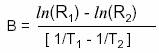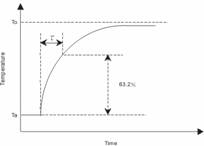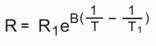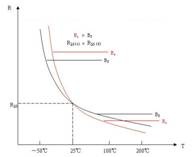﻿ Ntc thermistor technical parameters and calculation formula_

China sensor manufacturers

# Ntc thermistor technical parameters and calculation formula

NTC thermistor is a kind of metal oxide as the main raw material, After the high temperature sintering of the semiconductor ceramic temperature measurement resistance, Is a negative temperature coefficient resistance. Human through the resistance and B value of the law of change, Calculate the temperature. Yaxun Electronics provides the main parameters for the following parameters:
1. Actual resistance (RT)
Under certain temperature conditions measured resistance value.
2, zero power resistance (R25)
Generally refers to the ambient temperature of 25 ℃ when the actual resistance of the thermistor value.
3, B value
B value is a negative temperature coefficient thermistor thermal index. It is defined as the difference between the natural logarithm of the zero power resistance value at two temperatures and the difference from the reciprocal of the two temperatures.RT1 - zero power resistance at temperature T1
RT2 - zero power resistance at temperature T2
T1 = 2731.5k + (T1C), T2 = 2731.5k + (T2C)
Unless otherwise noted,The B value is calculated from the zero power resistance values of 25 ° C (298.15 K) and 50 ° C (323.15 K). The B value is not a strictly constant within the operating temperature range.
4, dissipation coefficient δ
δ = ΔP / ΔT (mW / ℃)
That is: at the specified ambient temperature, The ratio of the dissipated power change rate of the thermistor to its corresponding temperature change. It represents the power required to raise the temperature of the thermal energy resistor by 1 ° C.
In the operating temperature range, δ with changes in ambient temperature change.

5, the thermal time constant (τ)
Under zero power conditions, When the temperature mutation, Thermistor temperature changes the temperature difference of 63.2% of the required time. Τ is proportional to the heat capacity C of the thermistor, And its dissipation factor δ is inversely proportional to, that is:τ=C/δ
6, rated power (Pr)
Rated power = dissipation factor δ × (maximum operating temperature Tmax-25 ℃)

7, resistance - temperature characteristics
Thermistor resistance with the temperature changes in the law, as follows:
R1: Resistance at absolute temperature T1 (K)
B: B constant T (K) = T (° C) +273.15(1) B value is the same, the resistance is different              (2) the same resistance, B value is different
8, the switch temperature tb: PTC thermistor resistance value began to jump when the temperature.
9, dissipation coefficient H: the temperature increases 1 ℃, the thermistor dissipated power, the unit is mW / ℃.

﻿
﻿